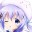Back

Db设计文档Melody 2019年11月24日

Typecho中抛弃了繁琐的MVC构建方式,将所有的功能单纯地封装为一个模块(也就是上述的Widget),使其更加适合开源地并发开发模式.由于没有了Model层的存在,Widget必须直接与数据库联系.Db类的设计就是为了帮助你更快速的获取自己所需要的数据。Typecho的Db对象使用方法与Zend Framework中的Db对象很相似，我们参考了Zend_Db中的Select对象组织方法，但不保证完全兼容。它们之间有一些差别，造成这些差别的原因之一就是Zend为不同的操作提供了不同的对象，而Typecho的所有操作都由Typecho_Db_Query来封装成SQL语句的，它的好处是你能在不同的操作中使用统一的API。

``````//get方法是Typecho_Db的静态方法
\$db = Typecho_Db::get();
``````

``````/** 定义数据库参数 */
\$db = new Typecho_Db('Mysql', 'typecho_');
'host'          =>  'localhost',
'port'          =>  '3306',
'user'          =>  'root',
'database'      =>  'typecho',
'charset'       =>  'utf8'
), Typecho_Db::READ | Typecho_Db::WRITE);
Typecho_Db::set(\$db);
``````

Typecho_Db内建的SQL语法构建器能够帮助你更容易地构建标准查询语句。

## SELECT查询语句

select是我们最常用的查询方式,以下代码向您展示了如何使用Db类从posts表中取出所有数据.

``````//获取实例化对象
\$db = Typecho_Db::get();

//获取查询语句对象
\$select = \$db->select()->from('posts');

//提交查询并获取结果
\$result = \$db->fetchAll(\$select);
``````

``````//重新构建select语句,在from方法中传递需要选择字段,并用半角逗号隔开
\$select = \$db->select('title', 'text', 'type')->from('posts');
``````

``````\$select = \$db->select('title', 'text', 'type')->from('posts')
->where('type = ?', 'post');
``````

``````\$select = \$db->select('title', 'text', 'type')->from('posts')
->where('type = ?', 'post')
->where('title = ?', 'hello');
``````

``````\$select = \$db->select('title', 'text', 'type')->from('posts')
->where('type = ? AND title = ?', 'post', 'hello');
``````

OR条件查询

``````\$select = \$db->select('title', 'text', 'type')->from('posts')
->where('type = ?', 'post')
->orWhere('type = ?', 'page');
``````

``````\$select = \$db->select()
->from('posts')
->where('type = ?', 'post')
->order('created', Typecho_Db::SORT_DESC);    //降序排序
``````

``````\$select = \$db->select()
->from('posts')
->where('type = ?', 'post')
->limit(10);    //仅取出10条记录
``````

``````\$select = \$db->select()
->from('posts')
->where('type = ?', 'post')
->offset(20)    //从第20条记录开始取
->limit(10);
``````

``````\$select = \$db->select()
->from('posts')
->where('type = ?', 'post')
->page(10, 20);    //按每页20条记录的方法取出第10页的记录
``````

``````\$select = \$db->select()
->from('posts')
->join('category', 'posts.category_id = category.id', Typecho_Db::LEFT_JOIN)
->where('`type` = ?', 'post');
``````

``````\$select = \$db->select('posts')
->from('posts');

//如果版本大于php5.2
echo \$select;

//如果小于php5.2
echo \$select->__toString();

//output: SELECT * FROM posts
``````

## INSERT语句

``````\$db = Typecho_Db::get();

\$insert = \$db->insert('post')
->rows(array('title' => 'example', 'content' => 'hello world'));

//将构建好的sql执行, 如果你的主键id是自增型的还会返回insert id
\$insertId = \$db->query(\$insert);
``````

INSERT INTO post (title, content) VALUES ('example', 'hello world')
DELETE语句
delete方法用于删除数据库中的制定方法。

``````\$db = Typecho_Db::get();

\$delete = \$db->delete('post')
->where('title = ?', example);

//将构建好的sql执行, 会自动返回已经删除的记录数
\$deletedRows = \$db->query(\$delete);
``````

DELETE FROM post WHERE title = 'example'

## UPDATE语句Submit